Next: Other Implications of Special Up: Special Relativity Previous: Length Contraction

# Lorentz Transformations and the Addition of Velocities

Time dilation and length contraction as given above describe how coordinates in two different frames are related for particular types of measurements. Time dilation occurs when observers compares the readings of two clocks in different frames of reference. Length contraction relates to the apparent decrease in the length of objects in motion relative to the observer.

We will now write down the general form of the transformation laws of Special Relativity that replace the Galilean transformation laws11.2 and 11.1 at high high relative velocities. They are:

 x =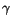x' +vt' (11.8) t =t' +x' (11.9)

where you will recall thatis the time dilation factor11.6. These remarkably simple formulas are called the Lorentz transformation laws. They contain virtually everything you need to know about special relativity. It is not necessary to study them in detail in order to understand the implications of Special Relativity. We write them down for two reasons. First, a cursory inspection will show that for low velocities, they are nearly identical to the Galilean transformation laws. Remember that if v is much less than c,is close to one. If you ignore, Eq.11.8 is identical to the Galilean equivalent 11.1. Moreover, the last term in 11.9 will be negligibly small when v is small, so that in this limit the Lorentz transformation equation gives t = t'. This correspondence with the Galilean transformation at low velocities had to be true for Special Relativity to be consistent with our everyday experience.

The second reason for writing down 11.8 and 11.9 is that they will allow us to deduce the expression for the addition of velocities in special relativity. We will do this in the same way that we derived the simple addition of velocities in the case of Galilean transformations. Let us return to Figs. 11.2 and 11.3 and consider a ball that is thrown in O' at a speed of u'. If the ball is thrown at t = t' = 0 and x = x' = 0 then in the O' frame the coordinate of the ball as a function of time is just x' = u't' where u' is the velocity relative to O'. The Lorentz transformation laws 11.8 and 11.9 tell us what the corresponding coordinates are in the unprimed frame. This immediately gives us the relationship between the velocities u'and u:

 u =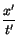==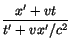(11.10)

Notice that the time dilation factor completely cancels out of the right hand side of the above equation. If we now divide the top and bottom by t', replace x'/t' by u' everywhere it occurs, we get the velocity transformation law:

 u =(11.11)

Eq.11.11 is an amazing formula. It differs from the common sense Galilean transformation law only by the second term in the denominator, but this extra term makes a world of difference for high relative velocities. However, if both u and v are small compared to c this term is negligibly small, and the formula reduces precisely to the Galilean transformation law 11.3 for the addition of velocities. However, for speeds that are significant compared to that of light, some very remarkable things happen.

For example, if instead of a ball, a beam of light is emitted by O', at a speed u' = c. What is the speed as measured in the frame O? In this case 11.11 gives

 u == c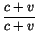= c (11.12)

As required by Special Relativity, the speed of light as measured in O is c, irrespective of the speed with which O' is moving.

We can also ask what happens if the frame O' is moving at three quarters the speed of light, and the ball is thrown at three quarters the speed of light in the same direction. What is the net velocity of the ball relative to O? Putting u = 3c/4 and v = 3c/4 into 11.11 we find

 u' =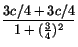=c =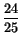c (11.13)

The net speed is still less than c! In fact it is easy to verify that no matter how fast O' is going (as long as it is less than c) and no matter how fast the ball is thrown (as long as it is less than c), the net velocity as measured relative to O will always be less than cas well.

There is yet another important implication of all this. In order to increase the velocity of an object you must exert a force over some finite distance. Thus, the agent exerting the force must to some extent be moving with the object that is being accelerated. We can approximate this process by a series of steps in which you accelerate the object instantaneously, then catch up to it and repeat the process. In particular, suppose you throw a ball at a speed of 3c/4, and then fire rockets so that you are moving along side. You then throw the ball again at 3c/4 relative to your new frame. The Galilean transformation law would imply that the ball is now moving relative to the initial frame at the 3c/2. However the relativistic law says that the ball is only moving at 24c/25 with respect to the initial frame. Since we are trying to approximate a process whereby you continuously accelerate the ball over a finite distance, you now fire your rockets again to catch up with the ball and then throw the ball one more time at 3c/4 relative to your new frame. In this case (v = 24c/25, 3c/4) the transformation law gives a velocity of relative to the initial frame of u = 171c/172. This is close, but still less than c. It should be clear at this stage, that no matter how often you repeat this process you will never get the ball to exceed the speed of light, although by repeating this process enough times you can get arbitrarily close. The implication is it is impossible to accelerate an object to speeds greater than that of light by exerting a finite force is over finite distance (i.e. by doing a finite amount of work). Thus, c is the ultimate speed limit in the Universe.Next: Other Implications of Special Up: Special Relativity Previous: Length Contraction
modtech@theory.uwinnipeg.ca
1999-09-29